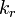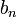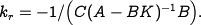# Errata: Formula for k r in equation (6.21) of Theorem 6.3 is incorrect

The equation forin the theorem is incorrect. The formula should bewhereis defined in equation (6.17). Alternatively,can be written as